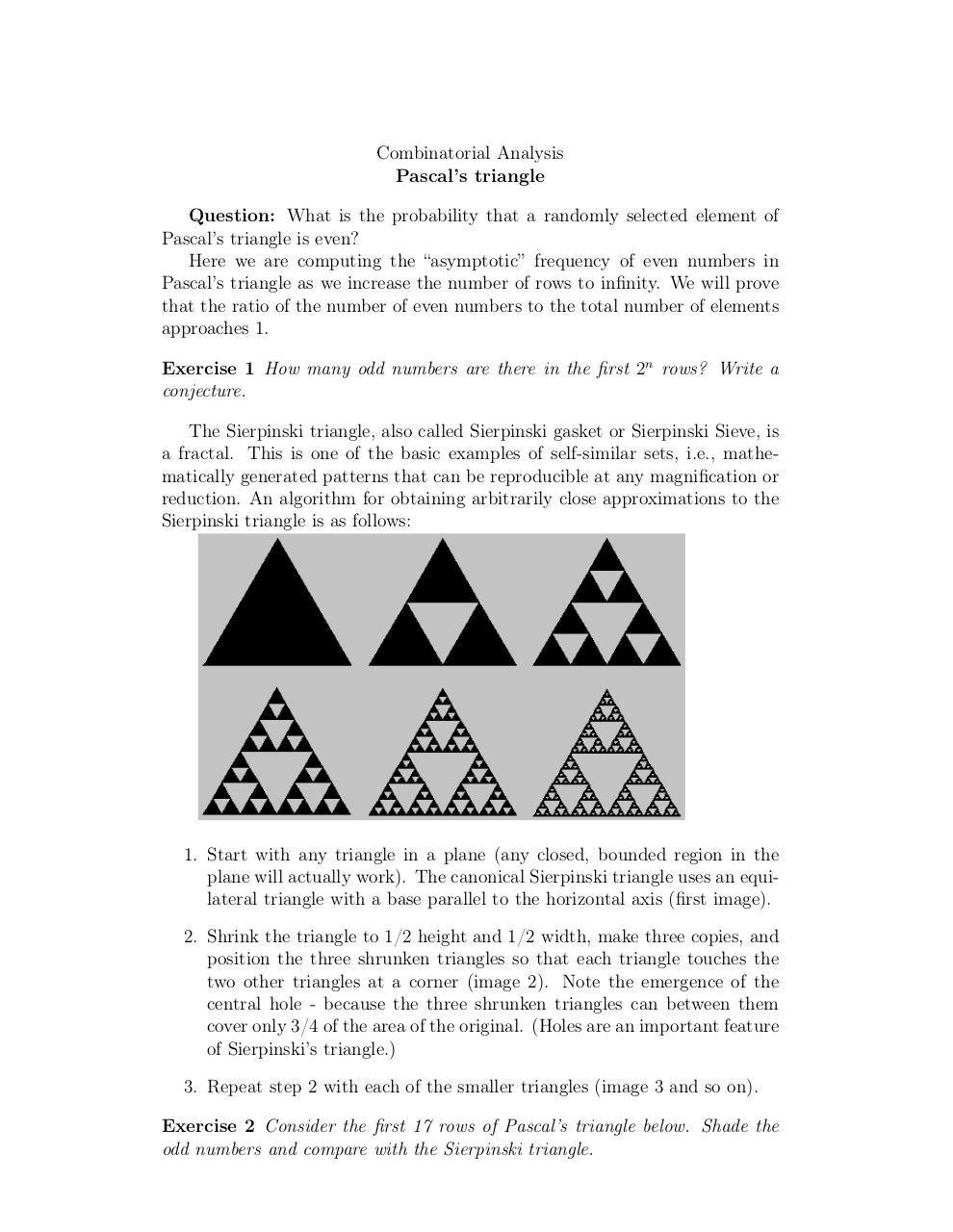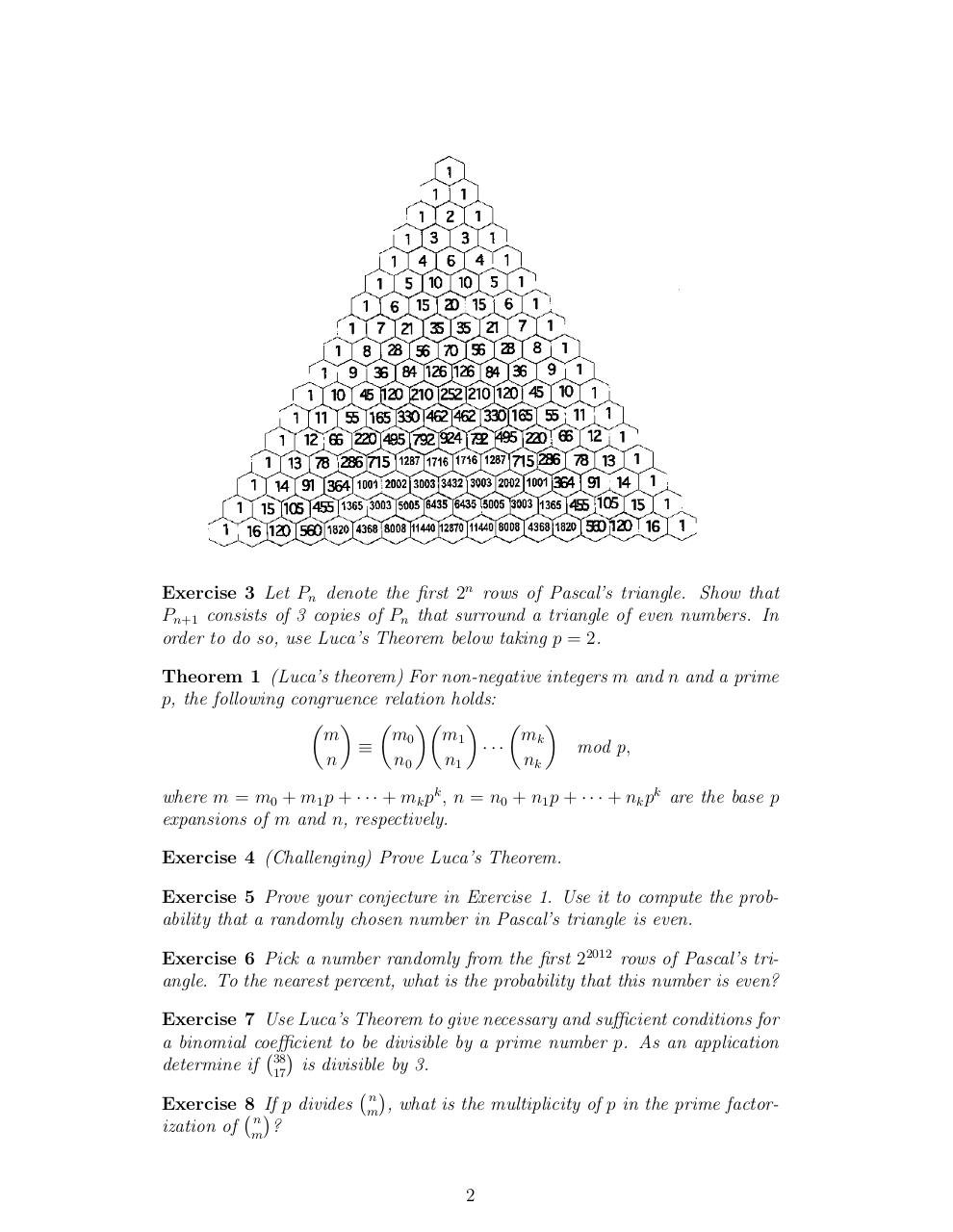# Day 12 Pascals triangle II .pdf

### File information

Original filename: Day 12-Pascals triangle II.pdf

This PDF 1.4 document has been generated by TeX / pdfTeX-1.40.3, and has been sent on pdf-archive.com on 24/01/2014 at 05:10, from IP address 169.231.x.x. The current document download page has been viewed 1232 times.
File size: 155 KB (2 pages).
Privacy: public file

Day 12-Pascals triangle II.pdf (PDF, 155 KB)

### Document preview

Combinatorial Analysis
Pascal’s triangle
Question: What is the probability that a randomly selected element of
Pascal’s triangle is even?
Here we are computing the “asymptotic” frequency of even numbers in
Pascal’s triangle as we increase the number of rows to infinity. We will prove
that the ratio of the number of even numbers to the total number of elements
approaches 1.
Exercise 1 How many odd numbers are there in the first 2n rows? Write a
conjecture.
The Sierpinski triangle, also called Sierpinski gasket or Sierpinski Sieve, is
a fractal. This is one of the basic examples of self-similar sets, i.e., mathematically generated patterns that can be reproducible at any magnification or
reduction. An algorithm for obtaining arbitrarily close approximations to the
Sierpinski triangle is as follows:

1. Start with any triangle in a plane (any closed, bounded region in the
plane will actually work). The canonical Sierpinski triangle uses an equilateral triangle with a base parallel to the horizontal axis (first image).
2. Shrink the triangle to 1/2 height and 1/2 width, make three copies, and
position the three shrunken triangles so that each triangle touches the
two other triangles at a corner (image 2). Note the emergence of the
central hole - because the three shrunken triangles can between them
cover only 3/4 of the area of the original. (Holes are an important feature
of Sierpinski’s triangle.)
3. Repeat step 2 with each of the smaller triangles (image 3 and so on).
Exercise 2 Consider the first 17 rows of Pascal’s triangle below. Shade the
odd numbers and compare with the Sierpinski triangle.

Exercise 3 Let Pn denote the first 2n rows of Pascal’s triangle. Show that
Pn+1 consists of 3 copies of Pn that surround a triangle of even numbers. In
order to do so, use Luca’s Theorem below taking p = 2.
Theorem 1 (Luca’s theorem) For non-negative integers m and n and a prime
p, the following congruence relation holds:
      
m
m0
m1
mk

···
mod p,
n
n0
n1
nk
where m = m0 + m1 p + · · · + mk pk , n = n0 + n1 p + · · · + nk pk are the base p
expansions of m and n, respectively.
Exercise 4 (Challenging) Prove Luca’s Theorem.
Exercise 5 Prove your conjecture in Exercise 1. Use it to compute the probability that a randomly chosen number in Pascal’s triangle is even.
Exercise 6 Pick a number randomly from the first 22012 rows of Pascal’s triangle. To the nearest percent, what is the probability that this number is even?
Exercise 7 Use Luca’s Theorem to give necessary and sufficient conditions for
a binomial coefficient
to be divisible by a prime number p. As an application

38
determine if 17 is divisible by 3.

n
Exercise 8 If p divides m
, what is the multiplicity of p in the prime factorn
ization of m
?

2#### HTML Code

Copy the following HTML code to share your document on a Website or Blog

#### QR Code### Related keywords